# 1 Introduction

The international IntCal group provides ratified radiocarbon calibration curves such as IntCal20 (for northern hemisphere terrestrial radiocarbon dates; Reimer et al. 20201), Marine20 (for marine dates; Heaton et al. 20202) and SHCal20 (Hogg et al. 20203). This package provides these curves, as well as previous iterations (IntCal13, Marine13, SHCal13, IntCal09, Marine09, IntCal04, Marine04, SHCal04, IntCal98, Marine98) and postbomb curves (Levin and Kromer 20044, Santos et al. 20155, Andrews et al. 20166, Hua et al. 20217). Also provided are functions to translate radiocarbon ages into different ‘realms’, as well as to quantify the impacts of contamination.

# 2 Installation

On first usage of the package, it has to be installed:

install.packages('rintcal')

If you have a recent version of rbacon, rplum or clam installed on your computer, rintcal will probably have been installed as well. Sometimes new versions of these packages appear, so please re-issue the above command regularly to remain up-to-date, or use:

update.packages()

library(rintcal)

# 3 Calibration curves

Now you can load a calibration curve into the memory, for example the default curve IntCal20, and check the first and last few entries:

ic20 <- ccurve()
head(ic20)
##   V1  V2 V3
## 1  0 199 11
## 2  1 197 11
## 3  2 195 11
## 4  3 193 11
## 5  4 190 11
## 6  5 188 11
tail(ic20)
##         V1    V2   V3
## 9496 54900 50009  997
## 9497 54920 50027 1003
## 9498 54940 50043 1007
## 9499 54960 50063 1013
## 9500 54980 50081 1018
## 9501 55000 50100 1024

The files have three columns: cal BP, the corresponding IntCal C14 BP ages, and the uncertainties (1 standard deviation).

To see more detail of each rintcal function, place a question-mark before the function name, e.g.:

?ccurve

To get a list of available curves and associated files (and where they can be found):

list.ccurves()
## /tmp/Rtmpl2bRdm/Rinst18607565c4ac9/rintcal/extdata/
##   "3Col_intcal04.14C"          "3Col_intcal09.14C"
##   "3Col_intcal13.14C"          "3Col_intcal20.14C"
##   "3Col_intcal98.14C"          "3Col_marine04.14C"
##   "3Col_marine09.14C"          "3Col_marine13.14C"
##   "3Col_marine20.14C"          "3Col_marine98.14C"
##  "3Col_shcal13.14C"           "3Col_shcal20.14C"
##  "Arnold_Libby_1951.txt"      "Kure.14C"
##  "LevinKromer.14C"            "Santos.14C"
##  "intcal20_data.txt"          "intcal20_data_sources.txt"
##  "postbomb_NH1.14C"           "postbomb_NH1_2009.14C"
##  "postbomb_NH1_monthly.14C"   "postbomb_NH2.14C"
##  "postbomb_NH2_2009.14C"      "postbomb_NH2_monthly.14C"
##  "postbomb_NH3.14C"           "postbomb_NH3_2009.14C"
##  "postbomb_NH3_monthly.14C"   "postbomb_SH1-2.14C"
##  "postbomb_SH1-2_2009.14C"    "postbomb_SH1-2_monthly.14C"
##  "postbomb_SH3.14C"           "postbomb_SH3_2009.14C"
##  "postbomb_SH3_monthly.14C"   "shcal20_data.txt"
##  "shcal20_data_sources.txt"

### 3.1.1 Libby’s curve

Legacy calibration data such as Arnold & Libby 1951 (Science 113, p. 111-120) can be compared with the latest calibration curves. Only included here are those radiocarbon dates of Arnold and Libby which come with independent (historical, archaeological or dendrochronological) age estimates. Note that Arnold and Libby did not define their ‘calendar years’ explicitly, so the ages have to be taken with a degree of caution.

libby <- read.table(file.path(system.file(package = 'rintcal'), "extdata/Arnold_Libby_1951.txt"), header=T, sep=",")
plot(libby[,2], libby[,4], xlab="'cal' BP", ylab="C14 BP") # plot the radiocarbon dates and their known calendar ages
segments(libby[,2]-libby[,3], libby[,4], libby[,2]+libby[,3], libby[,4]) # calendar error bars (not all are quantified)
segments(libby[,2], libby[,4]-libby[,5], libby[,2], libby[,4]+libby[,5]) # radiocarbon error bars
abline(0, 1, lty=2)
draw.ccurve(0, 10e3, add=TRUE) # add the most recent calibration curve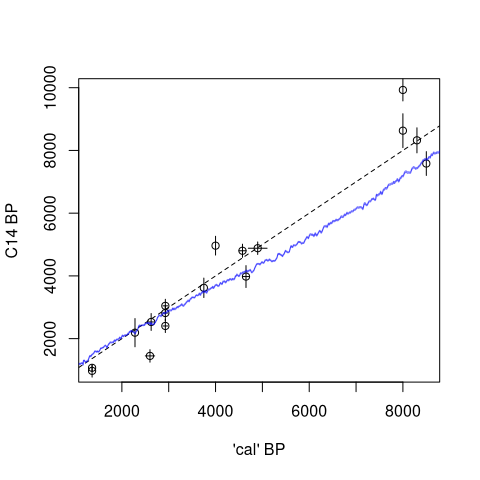## 3.2 Curve data

To look at the data underlying the IntCal curves, we can open the IntCal20 dataset downloaded from intchron.org and extract any relevant information, for example to check how many Irish oaks are in the dataset:

intcal <- intcal.read.data()
IrishOaks <- intcal.data.frames(intcal, taxon="Quercus sp.", country="Ireland")
## $taxon ##  "Quercus sp." ## ##$country
##  "Ireland"
length(IrishOaks)
##  17

Or plot a Bristlecone Pine series, one with single-ring radiocarbon dates that show a very abrupt change in radiocarbon age (Miyake Event):

Bristle <- intcal.data.frames(intcal, taxon="Pinus longaeva")
## $taxon ##  "Pinus longaeva" Bristle_yearly <- Bristle[]$data[,c(8,14,15)]
plot(Bristle_yearly[,1], Bristle_yearly[,2], xlab="cal BP", ylab="C14 BP")
segments(Bristle_yearly[,1], Bristle_yearly[,2]-Bristle_yearly[,3], Bristle_yearly[,1], Bristle_yearly[,2]+Bristle_yearly[,3])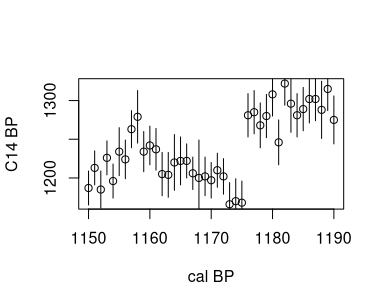## 3.3 Manipulations

You can also combine calibration curves, e.g. a 40%:60% mix of Intcal20 and Marine20 with a 100+-20 year offset for the latter. The resulting curve will be saved with the name mixed.14C, in a folder together with the calibration curves. The name of this folder is listed, and it can be changed by specifying the option ‘ccdir’.

mix.ccurves(0.4, cc1="IntCal20", cc2="Marine20", offset=cbind(100, 20))

To glue prebomb and postbomb calibration curves into one and store it as a variable in your session (for example, IntCal20 and the NH1 postbomb curve):

glued <- glue.ccurves("IntCal20", "NH1")
plot(glued[1:650,1:2], xlab="cal BP", ylab="C-14 BP", pch=".")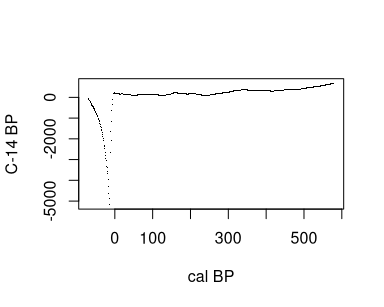## 3.4 Plots

We can also plot a calibration curve:

draw.ccurve()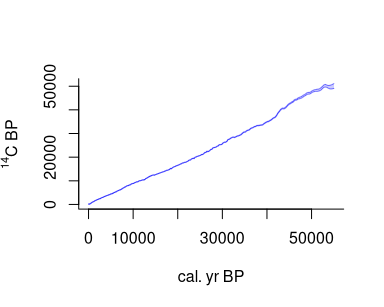Or compare two calibration curves:

draw.ccurve(1000, 2020, BCAD=TRUE, cc2='marine20', add.yaxis=TRUE)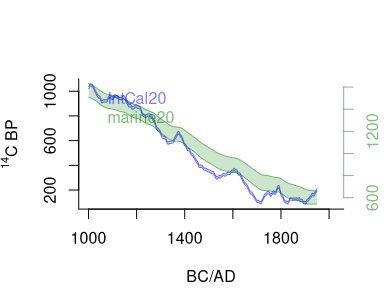Or zoom in to between AD 1600 and 2000 (using the BCAD scale):

draw.ccurve(1600, 1950, BCAD=TRUE)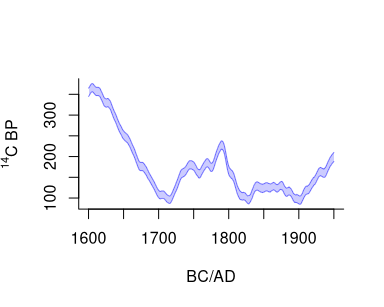Interesting things happened after 1950, as can be seen by adding a postbomb curve:

draw.ccurve(1600, 2020, BCAD=TRUE, cc2='nh1')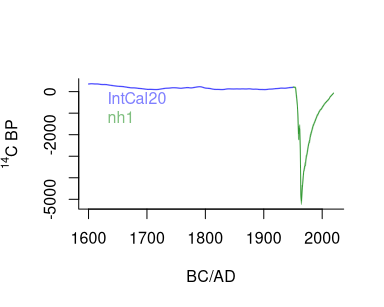The postbomb curve dwarfs the IntCal20 curve, so we could also plot both on separate vertical axes:

draw.ccurve(1600, 2020, BCAD=TRUE, cc2='nh1', add.yaxis=TRUE)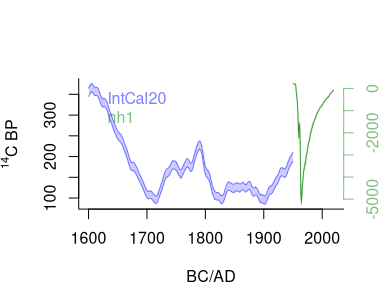We can also visualise the data underlying parts of the IntCal calibration curve, for example from 500 to 0 cal BP:

intcal.data(0, 500)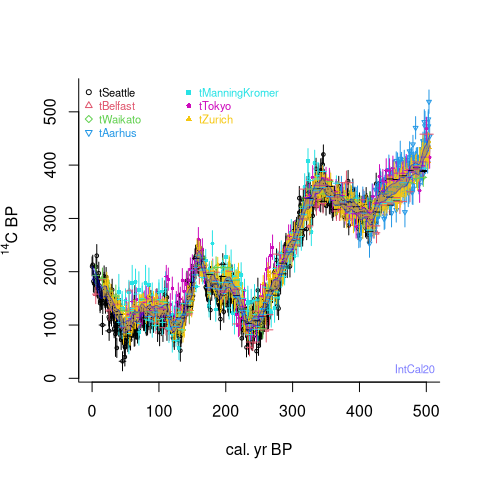Want to plot only some specific datasets over a period of time?

dat <- intcal.data(20e3, 25e3)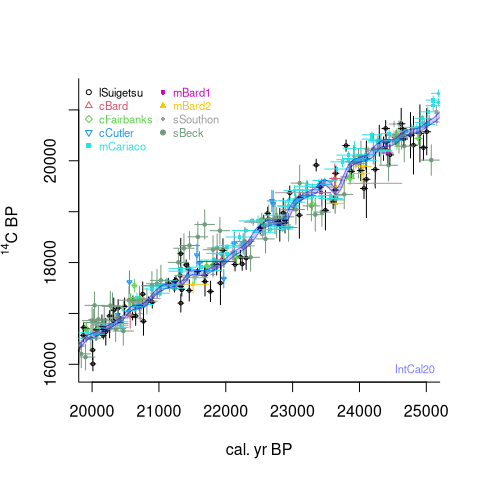unique(dat\$set)
##  109 110 111 114 120 121 122 124 126
dat <- intcal.data(20e3, 25e3, select.sets=c(109, 120), data.cols=c(1,2))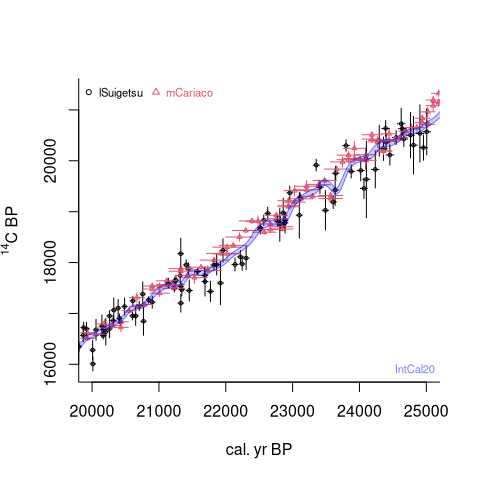# 4 Calculations

The rintcal package also provides some functions related to radiocarbon calibration. First there are two functions to calculate radiocarbon ages from pMC values (in this case of a postbomb date):

pMC.age(150, 1)
##  -3257    53

and the other way round:

age.pMC(-2300, 40)
##           y    sdev
## [1,] 133.15 0.66138

The same for calculations in the F14C realm:

F14C.age(.150, .01)
##          y   sdev
## [1,] 15240 518.44

and the other way round:

age.F14C(-2300, 40)
##           y      sdev
## [1,] 1.3315 0.0066138

To transfer $$\Delta^{14}C$$ (a proxy for atmospheric 14C concentration at t cal BP) to F14C, and the other way around:

F14C.D14C(0.71, t=4000)
##  151.8406
D14C.F14C(152, 4000)
##  0.7100983

These functions can be used to investigate $$\Delta^{14}C$$ over time:

cc <- ccurve()
cc.Fmin <- age.F14C(cc[,2]+cc[,3])
cc.Fmax <- age.F14C(cc[,2]-cc[,3])
cc.D14Cmin <- F14C.D14C(cc.Fmin, cc[,1])
cc.D14Cmax <- F14C.D14C(cc.Fmax, cc[,1])
par(mar=c(4,3,1,3), bty="l")
plot(cc[,1]/1e3, cc.D14Cmax, type="l", xlab="kcal BP", ylab="")
mtext(expression(paste(Delta, ""^{14}, "C")), 2, 1.7)
lines(cc[,1]/1e3, cc.D14Cmin)
par(new=TRUE)
plot(cc[,1]/1e3, (cc[,2]+cc[,3])/1e3, type="l", xaxt="n", yaxt="n", col=4, xlab="", ylab="")
lines(cc[,1]/1e3, (cc[,2]-cc[,3])/1e3, col=4)
axis(4, col=4, col.axis=4)
mtext(expression(paste(""^{14}, "C kBP")), 4, 2, col=4)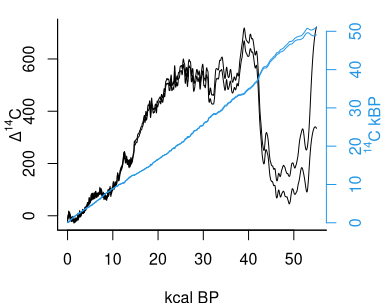## 4.1 Contamination

The above functions can be used to calculate the effect of contamination on radiocarbon ages, e.g. what age would be observed if material with a “true” radiocarbon age of 5000 +- 20 14C BP would be contaminated with 1% of modern carbon (F14C=1)?

contaminate(5000, 20, .01, 1)
##           y   sdev
## [1,] 4930.9 19.779

The effect of different levels of contamination can also be visualised:

real.14C <- seq(0, 50e3, length=200)
contam <- seq(0, .1, length=101) # 0 to 10% contamination
contam.col <- rainbow(length(contam))
plot(0, type="n", xlim=c(0, 55e3), xlab="real 14C age", ylim=range(real.14C), ylab="observed 14C age")
for(i in 1:length(contam))
lines(real.14C, contaminate(real.14C, c(), contam[i], 1, decimals=5), col=contam.col[i])
contam.legend <- seq(0, .1, length=6)
contam.col <- rainbow(length(contam.legend)-1)
text(50e3, contaminate(50e3, c(), contam.legend, 1),
labels=contam.legend, col=contam.col, cex=.7, offset=0, adj=c(0,.8))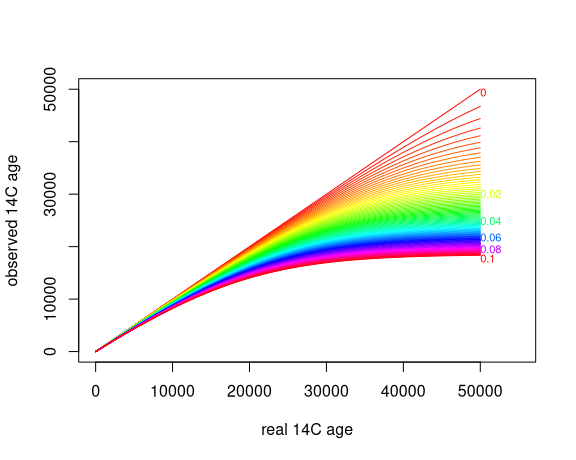If that is too much code for you, try this function instead:

draw.contamination()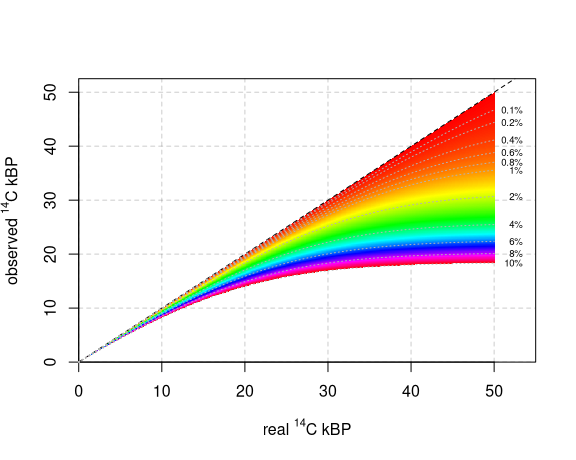## 4.2 Calibration details

Now on to calibration. We can obtain the calibrated probability distributions from radiocarbon dates, e.g., one of 130 +- 10 C14 BP:

calib.130 <- caldist(130, 10, BCAD=TRUE)
plot(calib.130, type="l")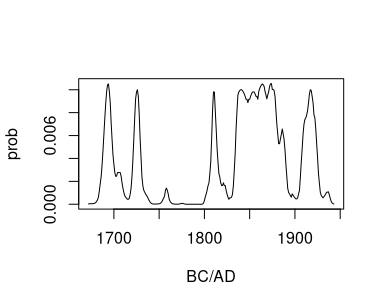For reporting purposes, calibrated dates are often reduced to their 95% highest posterior density (hpd) ranges (please report all, not just your favourite one!):

hpd(calib.130)
##      from   to perc
## [1,] 1685 1710 12.9
## [2,] 1719 1732  8.1
## [3,] 1758 1758  0.1
## [4,] 1804 1823  8.2
## [5,] 1832 1892 50.8
## [6,] 1906 1927 14.8

Additionally, calibrated dates are often reduced to single point estimates. Note however how poor representations they are of the entire calibrated distribution!

calib.2450 <- caldist(2450, 20)
plot(calib.2450, type="l")
points.2450 <- point.estimates(calib.2450)
points.2450
## weighted mean        median          mode      midpoint
##        2539.9        2512.3        2666.0        2531.5
abline(v=points.2450, col=1:4, lty=2)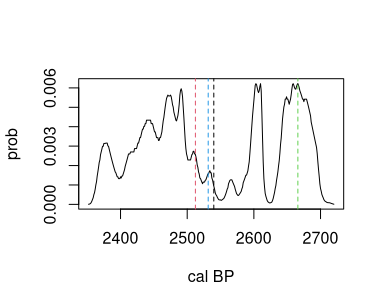Want a plot of the radiocarbon and calibrated distributions, together with their hpd ranges?

calibrate(130,10)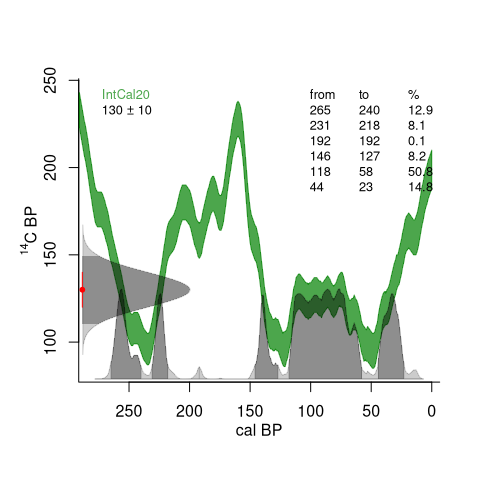## 4.3 Multiple calibrations

You can also draw one or more calibrated distributions:

set.seed(123)
dates <- sort(sample(500:2500,5))
errors <- .05*dates
depths <- 1:length(dates)
my.labels <- c("my", "very", "own", "simulated", "dates")
draw.dates(dates, errors, depths, BCAD=TRUE, labels=my.labels, age.lim=c(0, 1800))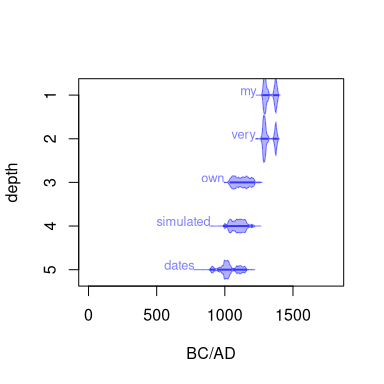or add them to an existing plot:

plot(300*1:5, 1:5, xlim=c(0, 1800), ylim=c(5,0), xlab="AD", ylab="dates")
draw.dates(dates, errors, depths, BCAD=TRUE, add=TRUE, labels=my.labels, mirror=FALSE)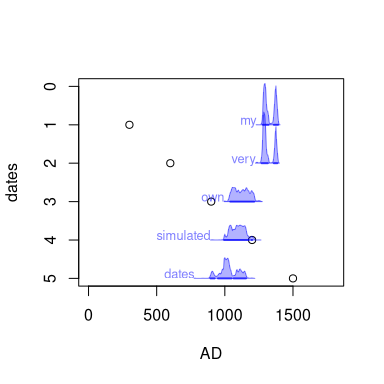or get creative (inspired by Jocelyn Bell Burnell8, Joy Division9 and the Hallstatt Plateau10):

par(bg="black", mar=rep(1, 4))
n <- 50; set.seed(1)
draw.dates(rnorm(n, 2450, 30), rep(25, n), n:1,
mirror=FALSE, draw.base=FALSE, draw.hpd=FALSE, col="white",
threshold=1e-28, age.lim=c(2250, 2800), ex=.8)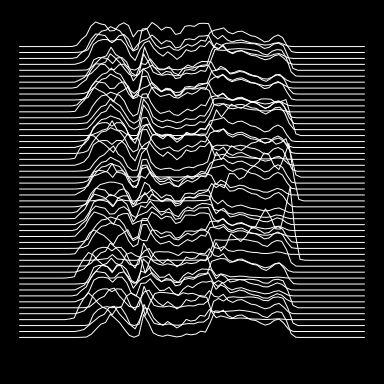1. Reimer PJ et al., 2020. The IntCal20 Northern Hemisphere radiocarbon age calibration curve (0-55 cal kBP). Radiocarbon 62, 725-757↩︎

2. Heaton TJ et al., 2020. Marine20-The Marine Radiocarbon Age Calibration Curve (0-55,000 cal BP). Radiocarbon 62, 779-820↩︎

3. Hogg AG et al., 2020. SHCal20 Southern Hemisphere Calibration, 0-55,000 Years cal BP. Radiocarbon 62, 759-778↩︎

4. Levin I, Kromer, B, 2004. The Tropospheric 14CO2 Level in Mid-Latitudes of the Northern Hemisphere (1959-2003), Radiocarbon 46, 1261-1272↩︎

5. Santos GM, Linares R, Lisi CS, Filho MT, 2015. Annual growth rings in a sample of Parana pine (Araucaria angustifolia): Toward improving the 14C calibration curve for the Southern Hemisphere. Quaternary Geochronology 25, 96-103↩︎

6. Andrews H, Siciliano D, Potts DC, DeMartini EE, Covarrubias S, 2016. Bomb radiocarbon and the Hawaiian Archipelago: Coral, otoliths and seawater. Radiocarbon 58, 531-548↩︎

7. Hua Q et al. 2021. Atmospheric Radiocarbon for the Period 1950-2019. Radiocarbon 64, 723-745↩︎